##### Statistics For Dummies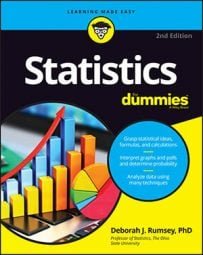The size (n) of a statistical sample affects the standard error for that sample. Because n is in the denominator of the standard error formula, the standard error decreases as n increases. It makes sense that having more data gives less variation (and more precision) in your results.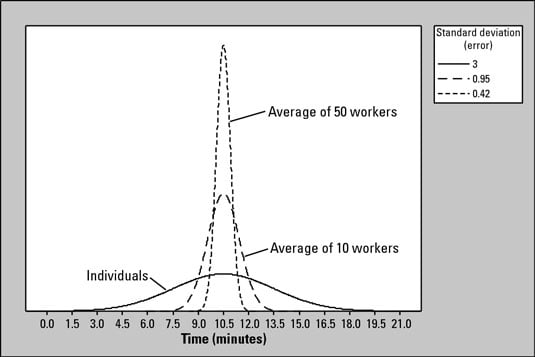Distributions of times for 1 worker, 10 workers, and 50 workers.

Suppose X is the time it takes for a clerical worker to type and send one letter of recommendation, and say X has a normal distribution with mean 10.5 minutes and standard deviation 3 minutes. The bottom curve in the preceding figure shows the distribution of X, the individual times for all clerical workers in the population. According to the Empirical Rule, almost all of the values are within 3 standard deviations of the mean (10.5) — between 1.5 and 19.5.

Now take a random sample of 10 clerical workers, measure their times, and find the average,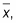each time. Repeat this process over and over, and graph all the possible results for all possible samples. The middle curve in the figure shows the picture of the sampling distribution of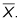Notice that it’s still centered at 10.5 (which you expected) but its variability is smaller; the standard error in this case is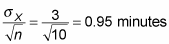(quite a bit less than 3 minutes, the standard deviation of the individual times).

Looking at the figure, the average times for samples of 10 clerical workers are closer to the mean (10.5) than the individual times are. That’s because average times don’t vary as much from sample to sample as individual times vary from person to person.

Now take all possible random samples of 50 clerical workers and find their means; the sampling distribution is shown in the tallest curve in the figure. The standard error of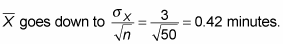You can see the average times for 50 clerical workers are even closer to 10.5 than the ones for 10 clerical workers. By the Empirical Rule, almost all of the values fall between 10.5 – 3(.42) = 9.24 and 10.5 + 3(.42) = 11.76. Larger samples tend to be a more accurate reflections of the population, hence their sample means are more likely to be closer to the population mean — hence less variation.

Why is having more precision around the mean important? Because sometimes you don’t know the population mean but want to determine what it is, or at least get as close to it as possible. How can you do that? By taking a large random sample from the population and finding its mean. You know that your sample mean will be close to the actual population mean if your sample is large, as the figure shows (assuming your data are collected correctly).